# ISEE Lower Level Math : How to find the distributive property

## Example Questions

1 2 3 4 5 6 7 9 Next →

### Example Question #81 : Numbers And Operations

Multiply: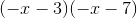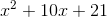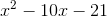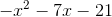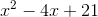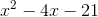Explanation:

To multiply these terms use the Distributive property by applying the FOIL method. The FOIL method requires that we multiply each term and then combine like terms: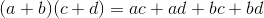Note: FOIL stands for First, Outside, Inside, Last, where

First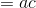Outside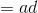Inside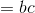Last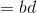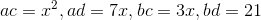Final solution equals:### Example Question #82 : Numbers And Operations

Evaluate: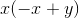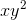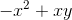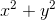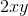Explanation:

To evaluate these terms apply the Distributive property: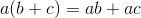Thus, the solution is: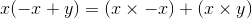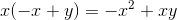Remember: we can not combine or add unlike variables or terms. For example,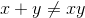### Example Question #83 : Numbers And Operations

Expand the expression.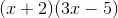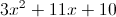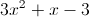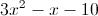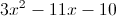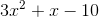Explanation:

Use FOIL (first, outer, inner, last) to expand.

First: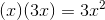Outside: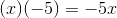Inside: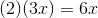Last: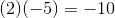Sum the four terms into one expression.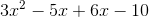Simplify by combining like terms.### Example Question #84 : Numbers And Operations

Jack purchasedtomato seeds and wants to make bags to sell at the local farmers’ market. How many different ways can Jack make seed bags with an equal number of seeds in each bag?Explanation:

We will solve this problem by finding factor pairs. Factor pairs are composed of two numbers that are multiplied together to equal a product. List all the factor pairs of Jack’s seeds.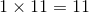Do not forget to list their reciprocals.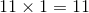Jack can makedifferent seed bag combinations with an equal number of seeds in each bag.

### Example Question #85 : Numbers And Operations

Jack purchasedtomato seeds and wants to make bags to sell at the local farmers’ market. If he wants to have at least two bags to sell, many different ways can Jack make seed bags with an equal number of seeds in each bag?Explanation:

We will solve this problem by finding factor pairs. Factor pairs are composed of two numbers that are multiplied together to equal a product. List all the factor pairs of Jack’s seeds.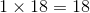-- but note that one bag of 18 seeds does not satisfy the condition that he wants to have at least two bags to sell, so this factorization does not count toward the answer.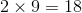-- two bags of nine seeds each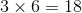-- three bags of six seeds each

Do not forget to list their reciprocals.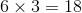-- six bags of three seeds each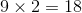-- nine bags of two seeds each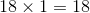-- eighteen bags of one seed each

Jack can make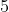different seed bag combinations with an equal number of seeds in each bag.

1 2 3 4 5 6 7 9 Next →